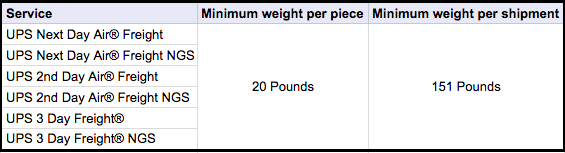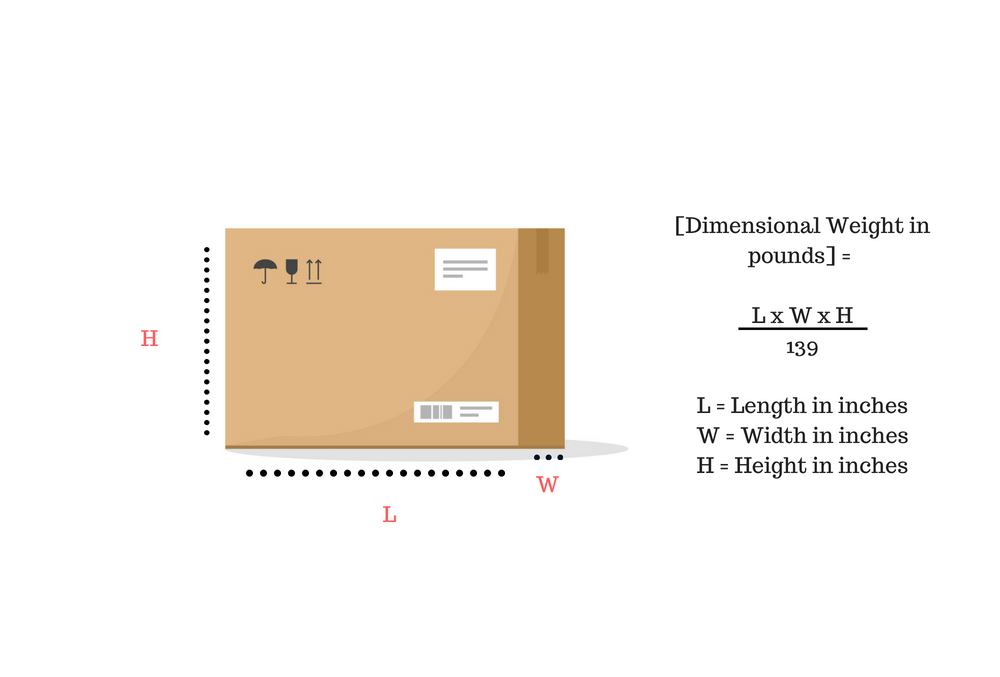# How billable weight is determined for UPS shipmentsWhen shipping with UPS, billable weight is the weight used to calculate the final rate. Years ago actual weight was used to calculate a final rate, but to improve efficiency billable weight was introduced. If shipping freight, you will need to calculate actual weight, billable weight, and dimensional weight to determine the billable weight. When the calculations are complete, the highest of the three is the billable weight.

## How to determine the billable weight

### 1. Determine the actual weight

The first step is to determine the actual weight. To find the actual weight, use a scale to weigh the package and round up to the next whole pound. For example, if your package weighs 10.65 pounds, you would round up to 11 pounds to find the actual weight.

### 2. Determine the minimum billable weight

If shipping freight or Large Packages, you will be subject to a minimum billable weight. Large Packages, with the exception of UPS Ground and Freight Pricing, have a minimum billable weight of 90 pounds – in addition to a Large Package Surcharge. Freight shipments follow the minimum billable weight outlined in the graph below.### 3. Determine the dimensional weight

In order to calculate the dimensional weight, you must first determine package dimensions. For each dimension, measure to the longest point and round up to the nearest whole number. Once each measurement is taken, multiply the package length, by the width and the height. The resulting measurement is the cubic size in inches. The next step is to divide the cubic size in inches by 139 to determine the dimensional weight in pounds. Increase any fraction to the next whole pound.

Example dimensional weight equation:

(L x H x W) / DIM
Ex. (7 x 9 x 12) / 139### 4. Determine billable weight

Now you will want to compare the actual weight, dimensional weight, and minimum billable weight. The greater of the three is designated as the billable weight and will be used to calculate the rate. For multiple-package shipments, calculate and total the billable weight of all packages in the shipment.

## Share a Refund experts are ready to help

Talk with a Share a Refund expert today about negotiating the best rates in your contract. Share a Refund contract optimization experts are ready to go to work for you to save you money and time.